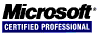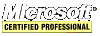## MFC HexDump Example Application (Microsoft Foundation Classes)

This MFC application opens a file to display its contents in a hexadecimal format. It is a simple application to learn the Microsoft Foundation Classes (MFC) Document/View Architecture. This code comes from Programming Windows with MFC Second Edition by Jeff Prosise. Compare it to the .NET HexDump. The meat of the program is listed below but the whole source can be found here: MFCHEXDUMP.zip

``````void CHexDumpView::FormatLine(CHexDumpDoc *pDoc, UINT nLine, CString &string)
{
UINT i;

// get 16 bytes and format them
BYTE b;
::FillMemory(b, 16, 32);
UINT nCount = pDoc->GetBytes(nLine * 16, 16, b);

string.Format(_T("%08X	 %0.2X %0.2X %0.2X %0.2X %0.2X %0.2X %0.2X %0.2X - %0.2X %0.2X %0.2X %0.2X %0.2X %0.2X %0.2X %0.2X	 "),
nLine * 16, b, b, b, b, b, b, b, b, b, b, b, b, b, b, b, b);

// replace non-printable chars with periods
for(i = 0; i < nCount; i++)
{
if(!::IsCharAlphaNumeric(b[i]))
b[i] = 0x2E;
}

// if less than 16 bytes were retrieved then erase to the end of line
b[nCount] = 0;
string += b;

if(nCount < 16)
{
UINT pos1 = 59, pos2 = 60;
UINT j = 16 - nCount;

for(i = 0; i < j; i++)
{
string.SetAt(pos1, _T(' '));
string.SetAt(pos2, _T(' '));
pos1 -= 3;
pos2 -= 3;
if(pos1 == 35)
{
string.SetAt(35, _T(' '));
string.SetAt(36, _T(' '));
pos1 = 33;
pos2 = 34;
}
}
}

}``````